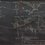# An open problem

My friend posed this question:

Does there exist an ordered pair $(a,b) \in \mathbb{Z}^{2}$ satisfying $a+b\pi = \pi^{\pi}$?

So far I've tried looking at properties of $\lbrace x\pi \rbrace-\lbrace \pi^{\pi} \rbrace$, at a few series representations of $\pi$, and at Mahler's classification of transcendental numbers (which I can't even begin to comprehend).

I hope someone more versed in linear algebra can provide a proof of $a+b\pi \neq \pi^{\pi}$, extending to $(a,b) \in \mathbb{Q}^{2}$.Note by Jake Lai
6 years, 7 months ago

This discussion board is a place to discuss our Daily Challenges and the math and science related to those challenges. Explanations are more than just a solution — they should explain the steps and thinking strategies that you used to obtain the solution. Comments should further the discussion of math and science.

When posting on Brilliant:

• Use the emojis to react to an explanation, whether you're congratulating a job well done , or just really confused .
• Ask specific questions about the challenge or the steps in somebody's explanation. Well-posed questions can add a lot to the discussion, but posting "I don't understand!" doesn't help anyone.
• Try to contribute something new to the discussion, whether it is an extension, generalization or other idea related to the challenge.

MarkdownAppears as
*italics* or _italics_ italics
**bold** or __bold__ bold
- bulleted- list
• bulleted
• list
1. numbered2. list
1. numbered
2. list
Note: you must add a full line of space before and after lists for them to show up correctly
paragraph 1paragraph 2

paragraph 1

paragraph 2

[example link](https://brilliant.org)example link
> This is a quote
This is a quote
    # I indented these lines
# 4 spaces, and now they show
# up as a code block.

print "hello world"
# I indented these lines
# 4 spaces, and now they show
# up as a code block.

print "hello world"
MathAppears as
Remember to wrap math in $$ ... $$ or $ ... $ to ensure proper formatting.
2 \times 3 $2 \times 3$
2^{34} $2^{34}$
a_{i-1} $a_{i-1}$
\frac{2}{3} $\frac{2}{3}$
\sqrt{2} $\sqrt{2}$
\sum_{i=1}^3 $\sum_{i=1}^3$
\sin \theta $\sin \theta$
\boxed{123} $\boxed{123}$

Sort by:

It is not known if $\pi ^{ \pi }$ is rational, algebraic or transcendental.

If such an ordered pair exists, then $\pi ^ { \pi }$ must be transcendental (since it is clear that $b \neq 0$).

Hence, there currently does not exist a proof if such an ordered pair exists.

Staff - 6 years, 7 months ago

Kind of related: Prove that if $a+b\sqrt{2}+c\sqrt{4}=1+\sqrt{2}+\sqrt{4}$ for $a,b,c\in \mathbb{Z}$, prove that $a=b=c=1$.

- 6 years, 7 months ago

When an integer $x$ is not a perfect cube, the vectors $x^{0}$, $x^{1}$ and $x^{2}$ are linearly independent? I don't know how one would go about proving that.

- 6 years, 7 months ago

An idea is substituting $a'=a-1$, $b'=b-1$, and $c'=c-1$. Then you just need to prove that given $a'+b'\sqrt{2}+c'\sqrt{4}=0$, prove that $a'=b'=c'=0$ which seems simpler to me.

Maybe then some casework, and we're done?

- 6 years, 7 months ago

Then you can rewrite as $b'\sqrt{2}+c'\sqrt{4}=-a'$ and cubing both sides, rearranging gives $-6b'c'\left(\sqrt{2}+\sqrt{4}\right)=a'^3+2b'^3+4c'^3$. Straightforward now.

- 6 years, 7 months ago

So we just need to prove that $\sqrt{2}+\sqrt{4}$ is irrational because $-\dfrac{a'^3+2b'^3+4c'^3}{6b'c'}$ is rational.

Suppose that $\sqrt{2}+\sqrt{4}$ is rational. Then $\dfrac{1}{1+\sqrt{2}+\sqrt{4}}=\sqrt{2}-1$ is also rational.

So $\sqrt{2}$ must be rational. So $\sqrt{2}=\dfrac{p}{q}$ for coprime positive integers $p,q$.

Cubing both sides, $2=\dfrac{p^3}{q^3}$ so $p^3=2q^3$ so $p$ is even.

Let $p=2p'$ so $8p'^3=2q^3$ so $4p'^3=q^3$ and $q$ is even.

But this contradicts our original assumption that $p,q$ are relatively prime so we are done.

- 6 years, 7 months ago